# Thunder and lightning

There was a glimpse of the sky, and we hear thunder in 4.5 seconds. The light spreads at 300,000 kilometers per second so that we can assume the flash instantly without delay. However, the sound's speed is much lower in the air, one-third of a kilometer per second. How far away is the storm?

s =  1.5 km

### Step-by-step explanation: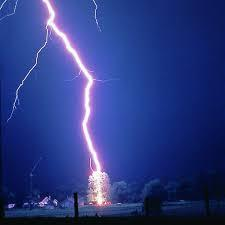Did you find an error or inaccuracy? Feel free to write us. Thank you!Tips to related online calculators
Do you want to convert length units?
Do you want to convert velocity (speed) units?
Do you want to convert time units like minutes to seconds?

## Related math problems and questions:

• Speed of sound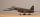The average speed of sound is 330 meters per second. Estimate how long it will take to hear the church bell 1 km away. Calculate the distance from what would hear sound after 10 seconds.
• Thunderstorm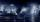The sound travels 1 km in about 3 seconds. How far is the storm if there is a time interval of 8 seconds between lightning and thunder?
• Sound speed in Earth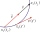Through the surface layers of the Earth, sound propagates 13 times faster than through air. During the geological survey, the charge exploded 1.7 km from the measuring station. How long does it take between the vibration of the soil at the measuring point
• Ping time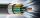Calculate theoretical ping time between Orlando and Shenzhen which is 14102 km distant. Ping time measures the round-trip time for small messages sent from the origin to a destination that is echoed back to the source. The name comes from active sonar ter
• Earth and Sun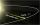Earth is 150 million km away from the Sun. 300,000 km of sunlight travels in 1 second. How long will the light come from the Sun to the Earth?
• Train delay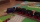Due to a breakdown, the train lost 16 minutes of standing on the track behind Brno. He "eliminated" this delay so that after the start, the 80 km long section went at a speed 10 km/h higher than originally planned. What speed was it and what was it suppos
• AbyssThe stone fell into the abyss: 2 seconds after we heard hitting bottom. How deep is the abyss (neglecting air resistance)? (gravitational acceleration g = 9.81 m/s2 and the speed of sound in air v = 343 m/s)
• The tourist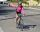The tourist came started from the hostel at an average speed of 5km/h. Half an hour later, the bicyclist started along the same route at a speed of 20km/h. How many minutes will a cyclist catch up and how many kilometers will he go?The railroad runs parallel to the railway. The train travels at 36 km/h on track. In the opposite direction, the motorcycle rides at 90 km/h. The train passes in 3 seconds. How long is a train?
• Two shipsThe distance from A to B is 300km. At 7 am started from A to B a ferry with speed higher by 20 km/h than a ship that leaves at 8 o'clock from B to A. Both met at 10h 24min. Determine how far they will meet from A and when they reach the destination.
• The Nürburgring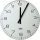The Nürburgring in Germany is a racetrack that hosts racing events all year. Two of the fastest laps ever driven on the track are 6 minutes 47.30 seconds and 6 minutes 52.01 seconds. How much faster is the 6 minutes 47.30 seconds time than the 6 minutes 5
• Two trains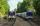Two trains go at each other. First at speed 40 km/h and the second at speed 60 km/h. At what distance will be 30 minutes before they will meet?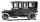Car started at 11:40 at speed 54 km/h from A and to B arrived at 12:20. Determine the distance A and B.Calculate how many minutes will be reduced to travel 187 km long railway corridor, where the maximum speed increases from 120 km/h to 160 km/h. Calculate how many minutes will shorten travel time, if we consider that the train must stop at 6 stations, eac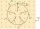When the cheetah began chasing the antelope, the distance between them was 120 meters. Although the antelope was running at 72km/h, the cheetah caught up with it in 12 seconds. What speed was the cheetah running?At 10 o'clock started from place A car speed at 90 km/h. Twenty minutes after starting another car from place B at a speed 60 km/h . Distance places A and B is 120 kilometers. What time and where do they meet?How long will fall airliner from a height of 10000 m at speed 1,000 km/h?